# Pyspark – Filter dataframe based on multiple conditions

• Last Updated : 04 Jul, 2021

In this article, we are going to see how to Filter dataframe based on multiple conditions.

Let’s Create a Dataframe for demonstration:

Attention geek! Strengthen your foundations with the Python Programming Foundation Course and learn the basics.

To begin with, your interview preparations Enhance your Data Structures concepts with the Python DS Course. And to begin with your Machine Learning Journey, join the Machine Learning - Basic Level Course

## Python3

 `# importing module``import` `pyspark`` ` `# importing sparksession from pyspark.sql module``from` `pyspark.sql ``import` `SparkSession`` ` `# creating sparksession and giving an app name``spark ``=` `SparkSession.builder.appName(``'sparkdf'``).getOrCreate()`` ` `# list  of students  data``data ``=` `[[``"1"``, ``"Amit"``, ``"DU"``],``        ``[``"2"``, ``"Mohit"``, ``"DU"``],``        ``[``"3"``, ``"rohith"``, ``"BHU"``],``        ``[``"4"``, ``"sridevi"``, ``"LPU"``],``        ``[``"1"``, ``"sravan"``, ``"KLMP"``],``        ``[``"5"``, ``"gnanesh"``, ``"IIT"``]]`` ` `# specify column names``columns ``=` `[``'student_ID'``, ``'student_NAME'``, ``'college'``]`` ` `# creating a dataframe from the lists of data``dataframe ``=` `spark.createDataFrame(data, columns)`` ` `# show dataframe``dataframe.show()`

Output: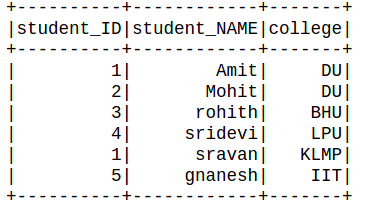### Method 1: Using Filter()

filter(): It is a function which filters the columns/row based on SQL expression or condition.

Syntax: Dataframe.filter(Condition)

Where condition may be given Logcal expression/ sql expression

Example 1: Filter single condition

## Python3

 `dataframe.``filter``(dataframe.college ``=``=` `"DU"``).show()`

Output: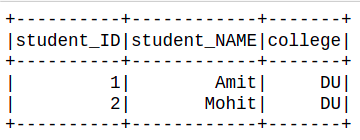Example 2: Filter columns with multiple conditions.

## Python3

 `dataframe.``filter``((dataframe.college ``=``=` `"DU"``) &``                 ``(dataframe.student_ID ``=``=` `"1"``)).show()`

Output: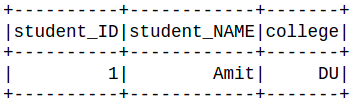### Method 2: Using filter and SQL Col

Here we are going to use the SQL col function, this function refers the column name of the dataframe with dataframe_object.col.

Syntax: Dataframe_obj.col(column_name).

Where, Column_name is refers to the column name of dataframe.

Example 1: Filter column with a single condition.

## Python3

 `# Using SQL col() function``from` `pyspark.sql.functions ``import` `col``dataframe.``filter``(col(``"college"``) ``=``=` `"DU"``).show()`

Output: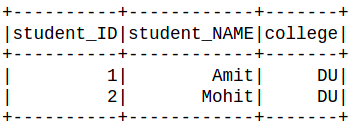Example 2: Filter column with multiple conditions.

## Python3

 `# Using SQL col() function``from` `pyspark.sql.functions ``import` `col`` ` ` ` `dataframe.``filter``((col(``"college"``) ``=``=` `"DU"``) &``                 ``(col(``"student_NAME"``) ``=``=` `"Amit"``)).show()`

Output: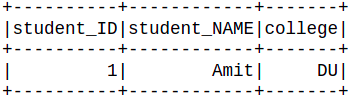### Method 3: Using isin()

isin(): This function takes a list as a parameter and returns the boolean expression. The boolean expression that is evaluated to true if the value of this expression is contained by the evaluated values of the arguments

Syntax: isin(*list)

Where *list is extracted from of list.

Example 1: Filter with a single list.

## Python3

 `list` `=` `[``1``, ``2``]``dataframe.``filter``(dataframe.student_ID.isin(``list``)).show()`

Output: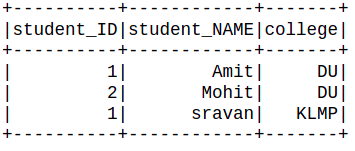Example 2: Filter with multiple lists.

## Python3

 `Id_list ``=` `[``1``, ``2``]``college_list ``=` `[``'DU'``,``'IIT'``]``dataframe.``filter``((dataframe.student_ID.isin(Id_list)) |``                 ``(dataframe.college.isin(college_list))).show()`

Output: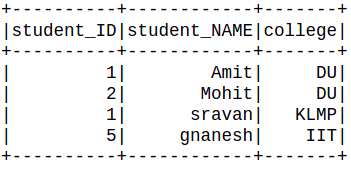### Method 4: Using Startswith and endswith

Here we will use startswith and endswith function of pyspark.

startswith(): This function takes a character as a parameter and searches in the columns string whose string starting with the first character if the condition satisfied then returns True.

Syntax: startswith(character)

Example:

## Python3

 `dataframe.``filter``(dataframe.student_NAME.startswith(``'s'``)).show()`

Output: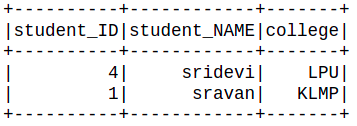endswith(): This function takes a character as a parameter and searches in the columns string whose string ending with the character if the condition satisfied then returns True.

Syntax: endswith(character)

Example:

## Python3

 `dataframe.``filter``(dataframe.student_NAME.endswith(``'t'``)).show()`

Output:Here will use both functions for filtering the dataframe:

## Python3

 `dataframe.``filter``((dataframe.student_NAME.endswith(``'t'``)) &``                 ``(dataframe.student_NAME.startswith(``"A"``))).show()`

Output:My Personal Notes arrow_drop_up